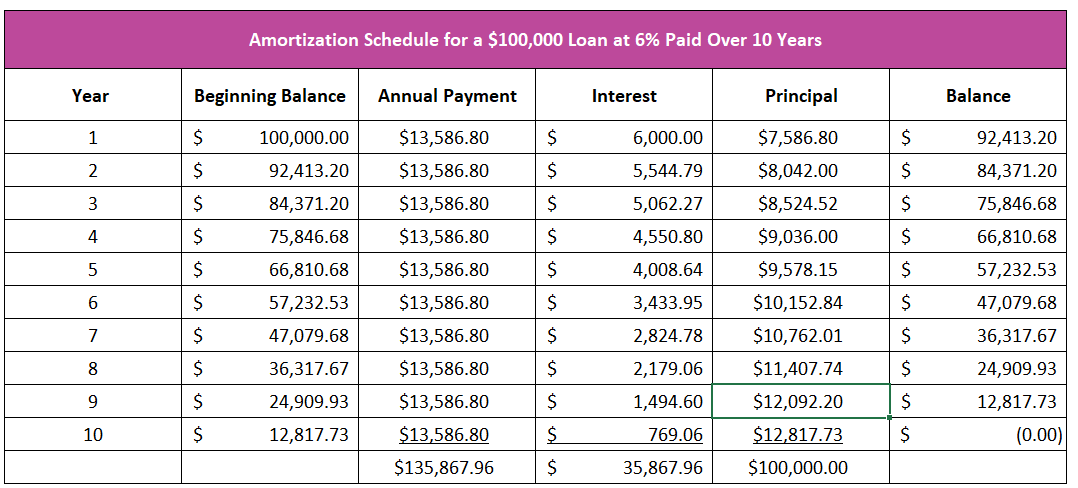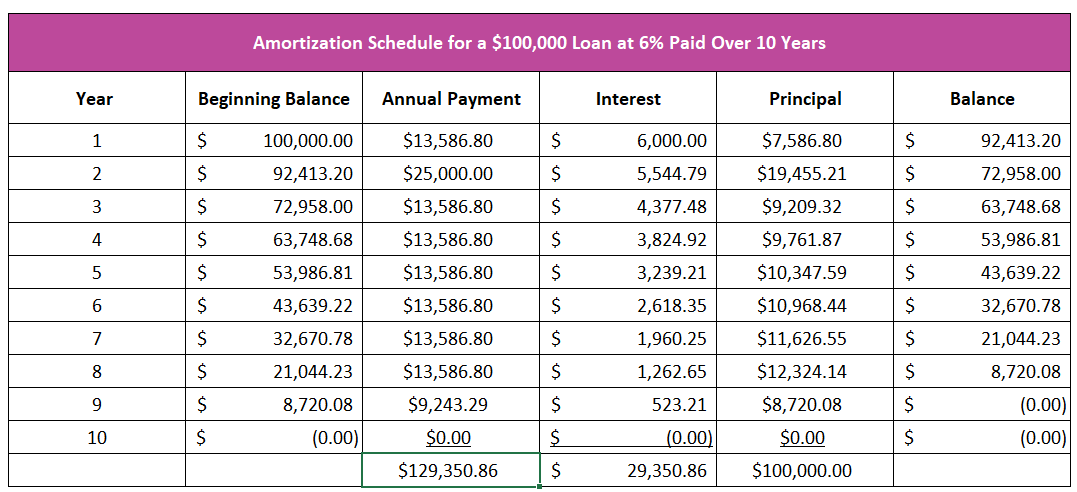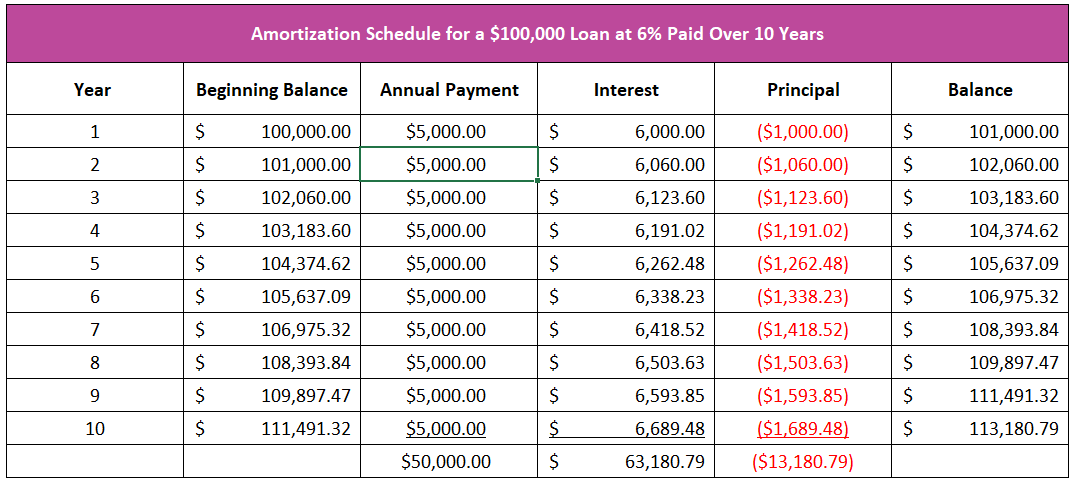# Compound Interest

## Definition of Compound Interest:

Compound interest is interest earning interest. The interest paid on the principal amount invested continues to compound because it earns interest when reinvested.

## Detailed Explanation:

To understand and fully appreciate the power of compound interest, it is helpful to compare two equal investments. One earns simple interest, while the other compound interest. Simple interest only pays interest on the principal invested. For example, bonds pay simple interest. A ten-year \$10,000 bond earning five percent annually, will pay \$500 (\$10,000*.05) each year until it matures. The principal investment remains \$10,000. Over ten years, the bond will earn \$5,000 interest.

An investment earning compound interest adds the interest in the preceding years to the initial investment, so the invested amount increases by the interest earned. That is what is meant by “interest earning interest.” For example, a ten-year CD that compounds at five percent annually will earn \$6,288.95 interest over the same ten years. Use the investment calculator to the left to illustrate. \$10,000 is the initial investment. The interest rate equals five percent. Only an initial \$10,000 investment is made, so the regular investment is left blank. The term is ten years, and the interest compounds annually. After calculating, you should see that the total value equals \$16,288.95 or the sum of your initial \$10,000 investment and \$6,288.95 interest. In the first year, the interest is \$500, or five percent of \$10,000 (the same as simple interest). However, in the second year, the original \$10,000 investment earns \$500, and the \$500 interest paid in the first year earns \$25 interest (\$500*.05), bringing the total value to \$11,025, or \$25 more than if the investment earned simple interest. After ten years, an investor would make \$1,288.95 more when the interest compounds annually.

Banks will frequently compound interest monthly or daily. A shorter time between compounding increases the return. In our example, switch compounded annually to monthly, and interest earned increases to \$6,470.09. Daily compounding yields an even higher return of \$6,493.42.

The mathematical formula for calculating the final value when interest is compounded annually is:

Future Value = P(1+i)n

Where P is the initial principal invested, i  is the interest rate, and n is the number of compounding periods.

Future Value = \$10,000 P(1+.05)10

The “rule of 72” is a quick way to calculate when an investment will double, assuming it compounds annually. Divide 72 by the interest rate (not expressed as a decimal) to determine the number of years required to double the investment. For example, assume a \$10,000 investment earns ten percent. The rule of 72 would estimate that the investment would grow to \$20,000 in 7.2 years. Using the calculator, we see that \$20,000 is reached in the seventh year.

Borrowers can be harmed or helped by the power of compounding when paying off a loan. Understanding an amortization schedule helps clarify how debt works and its dangers. The amortization schedule is a table showing the allocation of any payment to interest and principal. Borrowers must pay the interest first. The interest is the price for the use of money, so the larger the loan balance, the greater the interest. In our example, we assume a ten-year loan of \$100,000 with an interest rate of six percent. To simplify, assume one annual payment of \$13,586.80 and interest compounds annually. Table 1 is the amortization schedule for this loan. Notice that more interest is paid in the early stages of a loan. Any payment is the sum of the principal and interest, where interest is paid first. During the first year, \$6,000 in interest is paid (.06*\$100,000). Since interest is paid first, \$6,000 is subtracted from the payment amount, and the remainder reduces the principal balance. After the first payment, the principal reduction would equal \$7,586.80, or the difference between the \$13,586.80 payment and \$6,000 in interest. The end-of-year balance equals \$92,413.20 or the difference between \$100,000 and \$7,586.80. The allocation to principal and interest would be \$9,042 to principal and \$5,544.79 to interest after the second payment of \$13,586.80. The interest cost is lower because interest is only charged on the remaining balance of \$92,415.20.

Table 1Table 2 illustrates how a borrower may pay off a loan sooner and save money by making payments greater than the minimum required. Assume the second-year payment equals \$25,000. The interest paid is less each month, which results in the loan being paid off sooner. The higher payment saves much more than the additional principal paid since it negates the cost of future interest on any reduction in the loan balance. The borrower would save \$6,517.10.

Table 2Many people and businesses have learned a hard lesson in compounding when they secured a negatively amortizing loan. Negative amortization occurs when the payment is insufficient to cover the interest owed. Compounding hurts the borrower. Like any loan, interest is paid first, so since a payment is less than the interest, the owed interest is added to the loan balance. Table 3 below illustrates how a borrower ends up owing more than the original loan when payments do not cover the interest. The same loan is secured, but this time the borrower only pays \$5,000 each month. At the end of ten years, the borrower owes \$113,180.79.

Table 3